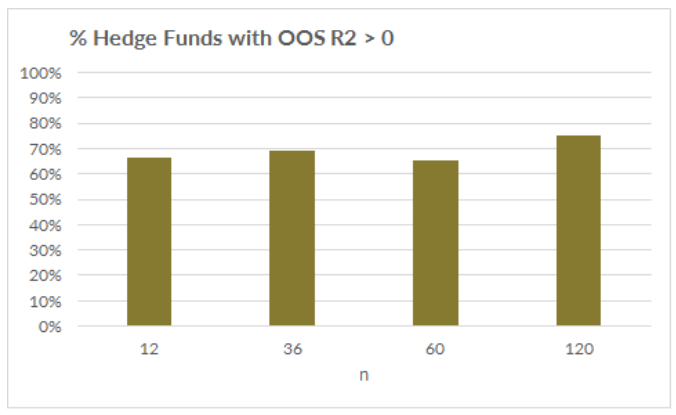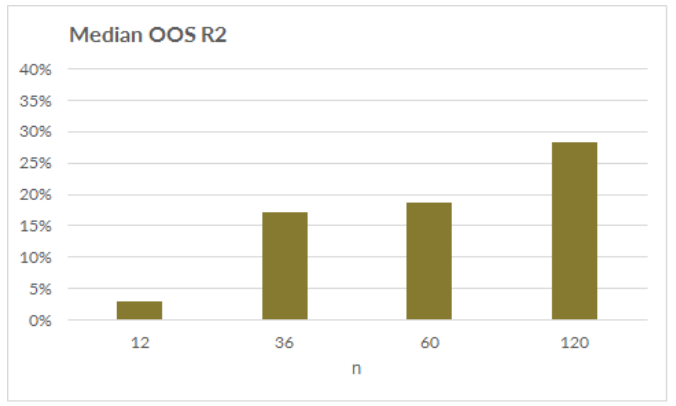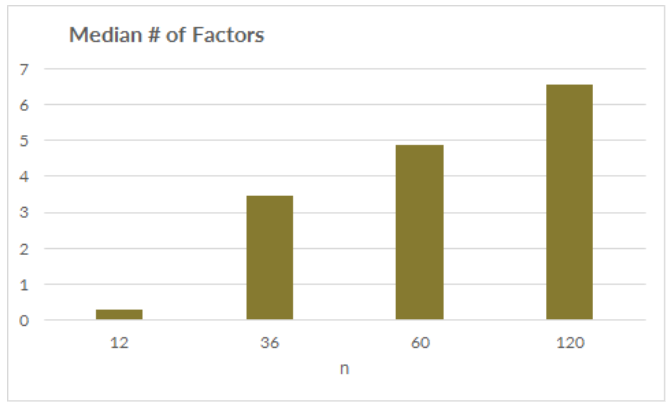## Introduction

The research team at Two Sigma that supports the Two Sigma Factor Lens as well as Venn’s more complicated analyses, like Scenario Analysis, spends a lot of time researching how Venn’s output can be more powerful for our subscribers. When Venn releases or updates a new feature, factor, or methodology, there can be days to months of research behind it. This holds true for even the seemingly smallest changes. To provide insight into the process, we will showcase some of the research that underpinned a recent change to Venn’s data requirements for Factor Analysis: reducing the minimum number of monthly return observations from 36 to 12.

## What Changed

As background, Venn uses regression analysis to determine an object’s (e.g., an investment’s or portfolio’s) relationship to the factors in the Two Sigma Factor Lens. Previously, the object was required to have at least 36 data points for monthly analysis. This presented a challenge for many subscribers who didn’t have three  years of return history for every investment in their portfolio. It left these subscribers with two options: proxy the short-history investments or remove them from the portfolio. With this challenge in mind, we explored options for reducing the minimum while preserving statistical robustness. As of April 30th, we updated those data requirements

While we still prefer more data to less for Factor Analysis, we are shortening the absolute minimum requirement to 12 monthly data points.1 This update also holds for Venn’s more complex, factor-based analyses, such as Scenario Analysis, Hypothetical Drawdown Analysis, Venncast, Optimization, and forward-looking forecasts. Previously, these analyses relied on the last three years of monthly data to extract the object’s recent factor exposures, whereas subscribers can now run these analyses on objects with less data. However, if a longer return history is available, the analyses will still rely on up to three years of recent return data.

This change makes it easier than ever before to get value out of Venn!## A Glimpse into the Research

It’s important to us that our subscribers feel assured that they are receiving meaningful output from Venn that proves useful in their investment decision-making process. While changing the minimum data requirement for Factor Analysis wasn't a huge build, a lot of research, testing, and careful consideration preceded the change. We’ll walk you through a subset of that research.

The goal of Factor Analysis on Venn is to determine whether a stable relationship exists between the object being analyzed and a set of factors. The output is factor exposures, or beta estimates, which measure the sensitivities of an object to the factors in the Two Sigma Factor Lens. The smaller the number of observations used in the analysis, the larger the error around the beta estimates (and vice versa -- the larger the number of observations, the tighter the distributions will be around the beta estimates).2

The question is, how much can we relax the minimum number of observations required so that more subscribers can get value out of Venn without sacrificing statistical robustness? We researched this question using “out-of-sample r-squareds” (OOS R2s) as a determination of the analysis’ efficacy and found that there were solidly positive OOS R2s with only 12 observations, even for single-fund analysis.

Sample Research Set Up

We tested a set of approximately 300 hedge funds3 where the OOS R2 for a given fund is constructed as follows:

1. Step forward through time. Each month run Venn’s regression methodology4 using the previous n observations.
2. Use those factor betas to predict the return for the next month (which is not a part of the regressions in step 1).5
3. Compare the predicted return to the actual return for that month.
4. Roll everything forward a month (running new regressions using the previous n months and predicting the next month).5
5. At the end, for a given fund, you have a time series of predictions and corresponding actuals. Compute the OOS R2 of the predictions on the actuals by taking [1 – variance(errors) / variance(actuals)], where errors is the difference between actuals and predictions.

The OOS R2 will measure how much of the actual return variation can be explained by the predicted returns. A positive OOS R2 implies that the prediction can explain hedge fund returns out of sample.6 The higher the OOS R2, the better the fit.

Let’s get into the research results. We compare the OOS R2s across the hedge funds by varying the size of “n”, or the number of monthly return observations.7

The first chart shows the percent of hedge funds with positive OOS R2s. The majority of funds exhibited positive OOS R2s for all variants of n.What about the median OOS R2 across the funds? Chart two below suggests that as n grows the median OOS R2 increases. While n=12 resulted in the smallest median OOS R2, the median value was still positive, indicating a predictive relationship between the factor model and the fund’s actual returns on average. Another interesting observation is that n=36 and n=60 produced very similar median OOS R2s, suggesting that the predictive power was not materially affected by adding two more years of monthly data to the analysis on average.8Overfitting, or the error caused by a factor model that is fit to what happened in the past and doesn’t actually work well out of sample, is a valid concern with using a smaller number of data points. We found that the first step of Venn’s regression methodology, the Lasso regression (see footnote 4 for more information), mitigates this risk by discarding noisy, irrelevant factors. As shown in the third and final chart below, the Lasso might have the ability (or degrees of freedom) to select a handful of factors for larger n values. However, it naturally restricts itself to one factor or less, on average, for n=12, indicating that it is controlling for the fewer number of observations.## Conclusion

To sum up, we presented a subset of the research that went into determining the data requirement for Factor Analysis on Venn that we felt met our clients’ needs while also providing valid statistical results. Although we prefer more data to less, we believe that the value that subscribers will unlock from this change is high, and the results are still fairly robust when using a smaller number of data points. The statistical robustness can be preserved largely thanks to the first step in Venn’s regression methodology. As seen in the last chart, expect to see a smaller number of factors in the Factor Analysis results for objects with a shorter return history, all else equal, as Venn’s Lasso recognizes the need to use a smaller number of factors to reduce the risk of overfitting. We take great care in making even the smallest decisions that affect your analysis output on Venn.

REFERENCES

1Factor Analysis data minimums will remain the same for daily returning objects: 6 months.

2Venn takes into account the beta estimate errors by displaying the t-statistics on hover when viewing Venn’s Factor Analysis results.

3The source for the hedge fund return data was Lipper TASS. We performed similar tests on a set of mutual funds as well as other investments.

4Venn uses a two-step regression methodology to determine factor exposures. The first step is a Lasso regression, which is used for factor selection. Lasso’s regularization parameter is specified using Akaike information criterion with small sample correction (AICc) and a 1.5 coefficient. The small sample correction is especially relevant for Venn’s subscribers, as limited data and short track records are quite common in the institutional space. Higher AICc coefficients indicate a higher bar for a factor to be accepted; lower regularization parameters loosen the acceptance threshold. After Lasso, the second step is an Ordinary Least Squares regression using the factors that survive (i.e., those factors with non-zero betas) the Lasso regression.

5To be extra conservative, we skipped a month between the regression and the prediction. So the regression went through t, and was used to predict t+2.

6The OOS R2 can also be negative. In that case, the direction of the beta estimate is wrong, and the variation of the errors is greater than the variation of the actual returns. For example, imagine the true relationship between a fund and the Equity factor was +1. If the analysis predicted a negative Equity beta for that fund, that would be a terrible result, providing a poor estimate of actual returns and resulting in a negative OOS R2.

7We also tested different lasso formulations by varying the AICc coefficient. The results shown here are using an AICc coefficient of 1.5 (the same as Venn). See footnote 4 for more information.

8This result held true across the other test sets – for higher n values (36 and above), the surface was generally flatter.

This article may include discussion of investing in virtual currencies. You should be aware that virtual currencies can have unique characteristics from other securities, securities transactions and financial transactions. Virtual currencies prices may be volatile, they may be difficult to price and their liquidity may be dispersed. Virtual currencies may be subject to certain cybersecurity and technology risks. Various intermediaries in the virtual currency markets may be unregulated, and the general regulatory landscape for virtual currencies is uncertain. The identity of virtual currency market participants may be opaque, which may increase the risk of market manipulation and fraud. Fees involved in trading virtual currencies may vary.